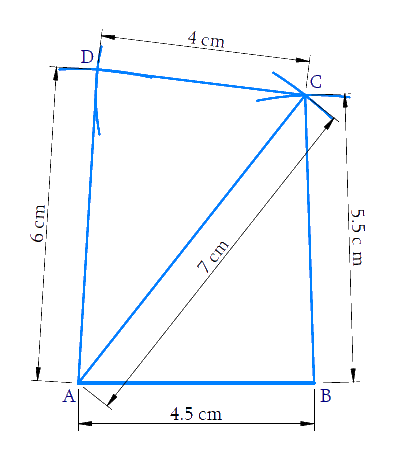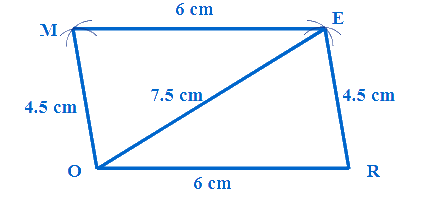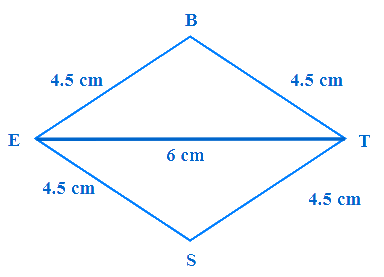NCERT Class 8 Maths Practical Geometry

# NCERT Class 8 Maths Practical Geometry

The chapter 4 deals with the various aspects of Practical Geometry and begins with an activity to do so.The procedure to construct a unique quadrilateral is discussed and the various cases involved are dealt individually.The construction of a unique quadrilateral when the lengths of four sides and a diagonal are given and when two diagonals and three sides are given are explained in detail.This is explained for other scenarios when two adjacent sides and three angles are known and when three sides and two included angles are given.Some special cases of construction are discussed in the last section of the chapter.

Download FREE PDF of Chapter-4 Practical Geometry

## Chapter 4 Ex.4.1 Question 1

Construct the following quadrilaterals.

(i) Quadrilateral $$ABCD.$$

$$AB = 4.5 \,\rm{cm}$$

$$BC = 5.5\,\rm{ cm}$$

$$CD = 4\,\rm{cm}$$

$$AD = 6\,\rm{cm}$$

$$AC = 7\,\rm{} cm$$

(ii) Quadrilateral $$JUMP$$

$$JU = 3.5\,\rm{cm}$$

$$UM = 4\,\rm{cm}$$

$$MP = 5\,\rm{cm}$$

$$PJ = 4.5\,\rm{cm}$$

$$PU = 6.5\,\rm{cm}$$

(iii) Parallelogram $$MORE$$

$$OR = 6\,\rm{cm}$$

$$RE = 4.5\,\rm{cm}$$

$$EO = 7.5\,\rm{cm}$$

(iv) Rhombus $$BEST$$

$$BE = 4.5\,\rm{cm}$$

$$ET = 6\,\rm{cm}$$

### Solution

What is the known?

Measurements of a Quadrilateral

What is unknown?

Construction of a Quadrilateral

Reasoning:

As you are aware, we need five measurements to draw a quadrilateral (Page 58 of Grade 8 NCERT Book)

Based on the given information, the five given measurements are of $$4$$ sides and one diagonal.When measurements of $$4$$ sides and one diagonal of a quadrilateral is given, we can construct a quadrilateral (Page 58 of Grade 8 NCERT Book)

Steps:

Let us first draw a rough sketch of quadrilateral.Construction of quadrilateral can be done in two parts. First construct triangle $$ABC$$ and then triangle $$ACD.$$ Let us find based on given measurements whether it is possible to construct the triangles.

In $$ABC,\;5.5+4.5>7$$ and $$5.5-4.5<7$$

$$7+4.5>5$$ and $$7-4.5<5.5$$

$$5.5+7>4.5$$ and $$7-5.5<4.5$$

It is possible to draw triangle $$ABC.$$

In $$ACD$$

$$7+4>6$$ and $$7-4<6$$

$$6+7>4$$ and $$7-6<4$$

$$6+4>7$$ and $$6-4<7$$

So construction of triangle $$ACD$$ is also possible.

Let us construct the quadrilateral.

Step 1: Draw line segment \begin{align}{{AB}} = 4.5\text{ cm}{{.}}\end{align} With $$B$$ as center and radius $$5.5\, \rm{cm}$$ draw an arc. With $$A$$ as center and radius $$7 \,\rm{cm}$$ draw another arc cutting the former arc. $$C$$ is the intersecting point of arcs. Join $$BC$$ and $$AC.$$

Step 2: Based on rough diagram, it is easy to find that $$AC$$ is the diagonal. Therefore, the fourth vertex $$D$$ should be on the opposite to $$B$$ with reference to $$AC$$.

Step 3: With $$A$$ as center and radius $$6\,\rm{cm}$$ draw an arc on the opposite side of point $$B.$$ With $$C$$ as center and radius $$4\,\rm{cm}$$ draw another arc cutting the former arc. $$D$$ is the intersecting point of the arcs. Join $$AD$$ and $$CD.$$ $$ABCD$$ is the required quadrilateral.

Related Problems.

Ex 4.1 (ii)

(iii) Parallelogram $$MORE$$

Using the concept opposite sides of parallelogram are parallel and equal, the five measurements can be found.(iv) Rhombus $$BEST$$

$$BE = 4.5\,\rm{cm}$$

$$ET = 6\, \rm{cm}$$

All sides of a rhombus are equal and opposite sides are parallel, measurements of all sides can be found.Practical Geometry | NCERT Solutions
Instant doubt clearing with Cuemath Advanced Math Program